Understanding

Cabinet Edge Diffraction

by

Andy Unruh,

Unruh Acoustics

Andrew_Unruh@excite.com

The phenomenon of cabinet edge diffraction is often poorly understood by amateur and professional loudspeaker system designers alike. I believe the root of the confusion is the natural tendency to try to explain and understand cabinet edge diffraction from the frequency domain point of view. Take the following quote taken from The LoudSpeaker Design Cookbook, 4th edition, written by Vance Dickason:

"Effectively, what is happening is the measurement circumstance is changing from free-field (4-pi) to half-space (2-pi) as the frequency increases and the wavelength decreases to something approaching the overall area of the baffle. This creates a response ‘step’ of about 6 dB, the frequency of the step being dependent upon the baffle area. The effect is most pronounced on-axis, as the baffle causes a beaming phenomenon like a [sic] -automobile headlight reflector."

While what is said is mostly correct, it does make one wonder:

• How does the wave know how large the baffle is?
• Why 6 dB?
• Are there any other effects?
• Are two baffles equivalent if they have the same area but vastly different dimensions, e.g. a 30 cm X 30 cm baffle vs. a 5 cm X 180 cm baffle?

In my opinion, the key to developing an intuitive or quantitative understanding of cabinet edge diffraction is by studying it primarily the time domain and resorting to the frequency domain only when absolutely necessary. Once the effect is understood in the time domain, it is easily translated to the frequency domain by using the Fourier transform.

Imagine an ideal point source hemispherical radiator mounted on the exact center of the end of a long cylindrical solid. Such a radiator will exhibit a hemispherical radiation pattern that is independent of frequency. In addition, let us suppose it exhibits minimum phase characteristics and has flat frequency response over all frequencies. If we now excite the radiator with a discrete-time impulse of duration 0.025 mS, it will move in response to the impulse and stimulate a hemispherical acoustic impulse moving away from the point source at the speed of sound. Since the radiator is perfect, the acoustic impulse will have a shape identical to the discrete-time impulse.

Everything is very easy to visualize until the edge of the impulse reaches the edge of the cylinder. When the impulse reaches the edge of the cylinder, there is a sudden loss of support as the impulse is now free to radiate behind the face of the cylinder, not just in front of it. In this way, the impulse ‘diffracts’ or ‘scatters’ behind the face of the cylinder. Interestingly, this scattering is frequency independent, but angle dependent. So if we measured the acoustic signal behind the cylinder, we would find that it is an impulse identical to the one formed by the radiator but somewhat diminished in magnitude. Now, few of us set up our favorite listening spot behind our loudspeakers, so it makes sense to try to understand what happens in front of the loudspeaker. Due to the loss of support at the edge of the cylinder, the impulse will partially collapse as some of the pressure ‘leaks’ backwards and this causes a secondary impulse to scatter in the forward direction. Like the impulse scattered behind the cylinder, the forward-scattered impulse will also be frequency independent but angle dependent. Unlike the backward-scattered impulse, though, the forward-scattered impulse will have the opposite polarity as the original impulse. Now imagine a microphone located in front of and on the cylinder axis, far away from the cylinder. What will the microphone measure? First, the impulse from the radiator will be picked up then, delayed by an amount equal to the radius of the cylinder divided by the speed of sound, the forward-scattered impulse will be measured.

Now, as stated previously, neither the forward nor the backward-scattered impulses display frequency dependence. However, taken together, the direct and forward-scattered impulses will result in frequency dependence through constructive and destructive interference. Figure 1 and Figure 2 show the time and frequency domain behavior of the impulse as measured by a microphone located in front of and on the cylinder axis, far away from the cylinder. The radius of the cylinder is 1 meter and the radiator is mounted in the center of the baffle.

It is important enough to reiterate – the frequency response of either the direct impulse (shown in Figure 1 at 0 seconds) or the diffracted impulse (shown in Figure 1 at 3 mS), taken alone is flat. It is only the combination of the direct and diffracted impulses, which leads to the complicated behavior shown in Figure 2.

How do the two impulses combine to form frequency response shown in Figure 2? The acoustic impulse contains all of the frequencies from DC to 20,000 Hz (1/2 of the sample rate), and has flat frequency response. When the direct impulse combines with the diffracted impulse, which is delayed by about 3 mS and of opposite polarity compared to the direct impulse, there will be frequencies that are reinforced and frequencies that are diminished. The maximums in the frequency response will occur where,

Frequency(N) = (N+0.5)/Delay. N = 0, 1, 2, 3, etc.

For a delay of 3 mS, peaks are predicted for frequencies of 167, 500, 833, 1167 etc. Hz.

The minimums in the frequency response will occur where,

Frequency(N) = N/Delay. N = 0, 1, 2, 3, etc.

For the same delay, dips are predicted for frequencies of 0, 333, 666, 999, etc. Hz.

It should be observed that the distance between peaks and dips is constant for the case of a circular baffle. They appear to get closer together at higher frequencies in Figure 2 only because of the log frequency scale that was used.

Now if the cylinder had a radius of only 1/3 meter instead of 1 meter, the diffracted impulse would arrive at the microphone sooner. This changes the time domain behavior and thus the frequency domain behavior. This is shown in Figures 3 and 4.

Comparing Figures 2 and 4, it is easily seen that, form a frequency response standpoint, the effect of reducing the baffle size is to move the oscillations in magnitude to higher frequencies and to increase the bandwidth of each oscillation, e.g. the oscillations are much closer together for a larger baffle size. The reason for this is easily seen by referring to either of the two above equations. Doing so immediately shows that 1/Delay Hz separates consecutive peaks (or consecutive dips).

Figure 5 and Figure 6 show the time and frequency domain behavior of the impulse as measured by a microphone located in front of and on the axis of a rectangular solid with a square baffle, far away from the end. These simulations were calculated with the radiator mounted in the exact center of the baffle. The area of the square baffle is the same as that of the cylinder whose radius was one meter. As can be seen in Figure 5, the direct impulse is identical to that shown in Figure 1, but the diffracted impulse is spread out over time and thus will be referred to as the diffracted signal. Interestingly, the sum of the total diffracted signal shown in Figure 5 is identical to the magnitude of the diffracted impulse shown in Figure 1. This means that a square baffle does not reduce the total amount of diffraction, it only spreads it out over time. This spread is simply due to the fact that the distance between the radiator and the edge of the cylinder was constant in Figure 1 while the distance between the radiator and the edge of the square baffle varies. By comparing Figures 2 and 6, an interesting result is noticed. While at low frequencies the results are very similar, in Figure 2, the oscillations in the magnitude are uniform while in Figure 6 they tend to decrease with frequency. The simple reason for this difference is that the Fourier transforms of the two time domain responses shown in Figures 1 and 5 are different. For those unsatisfied with this simple explanation, a somewhat more intuitive explanation is given. Imagine that instead of the excitation signal being an impulse, the excitation signal is a very low-frequency sinusoid. The sinusoid diffracts at the edge of the square just like the impulse and is similarly smeared in time. The time period over which it is smeared, however, is small compared to the sinusoid’s period, thus the contributions from each little part of the baffle to the diffracted sinusoid are essentially coherent and the diffracted sinusoid combines out of phase with the direct sinusoid. Next consider a high frequency sinusoid. At high frequencies, the time period over which the diffracted sinusoid is smeared is large compared to the sinusoid’s period thus the contributions to the diffracted sinusoid are essentially non-coherent and thus sum to a smaller value than in the low frequency case. Thus the diffracted sinusoid is of smaller magnitude and the total signal more fully dominated by the strength of the direct signal. In the case of the cylinder, the diffracted sinusoids are not smeared in time at all; thus the contributions to the diffracted sinusoid are always coherent.

Figure 7 and 8 show the time and frequency domain behavior of the impulse as measured by a microphone located in front of and on the axis of rectangular solid with a rectangular baffle, far away from the end. The radiator is placed in the center of the baffle. The area of the rectangular baffle is the same as that of the cylinder whose radius was one meter and the square baffle. The dimensions of the rectangular baffle are sqrt(pi)/4 X sqrt(pi)*4. Comparing Figures 5 and 7, one can see that while the diffracted signal is spread out in time for both, it is spread out over a much longer time in Figure 7. In Figure 5, the diffracted signal is concentrated around 3 mS, while in Figure 6 the diffracted signal has a large concentration around 0.7 mS and a smaller concentration around 11 mS. Though not visible in Figure 7, there is actually a continuous distribution of diffracted signal between about 0.7 mS and 11 mS. The large concentration of diffracted signal in Figure 7 corresponds to diffraction occurring on the left and right sides nearest the radiator; the smaller concentration corresponds to diffraction from the top and bottom of the baffle. The reason for the difference in the magnitudes of the two concentrations is simply that less of the direct signal intersects the top and bottom than the right and left sides of the baffle. The sum total of the diffracted signals in Figures 1, 3, 5, and 7 are all identical, they only differ in their distribution over time. Comparing Figures 6 and 8, it is easily seen that the so-called ‘baffle step’ occurs at a much higher frequency in Figure 8. Thus it is evident that the frequency at which the baffle step occurs is not strictly dependent upon the baffle area, but is instead dependent upon the dimensions of the baffle and the position of the radiator on the baffle. Later, it will be shown that the position of the baffle step is most strongly influenced by the minimum distance from the radiator to the baffle edge. There are both coarse and fine structures visible in Figure 8. The coarse structure is dominated by the first (larger) concentration of diffracted signal shown in Figure 7 while the fine structure is dominated by the second (smaller), concentration of diffracted signal. The nature of the coarse and fine structures is in keeping the observations shown in Figures 2 and 4 showing that the longer the delay between the direct signal and the diffracted signal, the closer the oscillations are to each other.

Figure 9 and Figure 10 show the time and frequency domain behavior of the impulse as measured by a microphone located in front of and on the axis of a rectangular solid with a square baffle, far away from the end. The dimensions of the baffle are the same as those referred to in Figures 5 and 6, but this time the radiator is placed in the upper left-hand corner of the baffle, only 0.1 meters from the top and left edges. Looking at Figure 9, it is seen that nearly the entire diffracted signal is clustered around 0.3 mS. This of course corresponds to the time required for the direct signal to reach the edge of the baffle, 0.1 meters away. Moving now to Figure 10, it is seen that the baffle step occurs at a very high frequency, despite the large baffle dimensions. As alluded to in the previous paragraph, the frequency associated with the baffle step is seen to most strongly depend on the minimum distance between the radiator and the edge of the baffle. Figure 10 also shows both coarse and fine structure. The reasons for this are the same as for Figure 8.

In summary the following was discussed and explained:

• The origin of the ‘baffle step’ and its dependence on baffle dimensions and transducer position
• The origin of the oscillations in the magnitude response of a transducer mounted on a baffle and its dependence on baffle dimensions and transducer position.
• The reason why certain ‘magic numbers’ work to reduce the magnitude of the oscillations of a transducer mounted on a baffle.
• The differences between an ideal point source hemispherical radiator and a real transducer on cabinet edge diffraction.

The following was not explained:

• The root cause of diffraction
• The dependence of diffraction magnitude on angle

For further information, I suggest that the interested reader obtain a copy of Reference II through the Audio Engineering Society at www.aes.org.

References:

I. Dickason, Vance, "The Loud Speaker Design Cookbook", 4th edition, Audio Amateur Press, Peterborough, New Hampshire., p. 77

II. Vanderkooy, John, "A Simple Theory of Cabinet Edge Diffraction", JAES, Vol. 39, pp. 923-933

Figure 1. Time domain behavior of a point source mounted in the center of the face of a long cylinder. Cylinder has a radius of 1 meter and measurement is taken far from the cylinder face.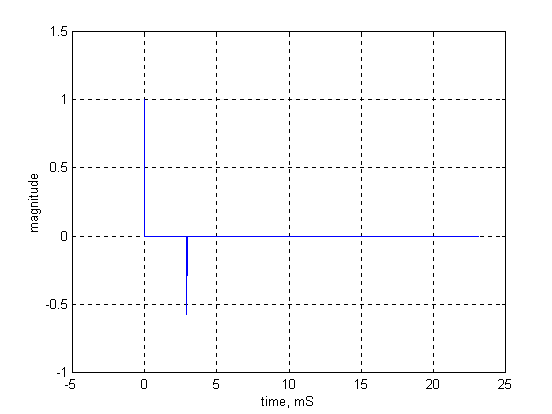Figure 2. Frequency domain behavior of a point source mounted in the center of the face of a long cylinder. Cylinder has a radius of 1 meter and measurement is taken far from the cylinder face.Figure 3. Time domain behavior of a point source mounted in the center of the face of a long cylinder. Cylinder has a radius of .33 meter and measurement is taken far from the cylinder face.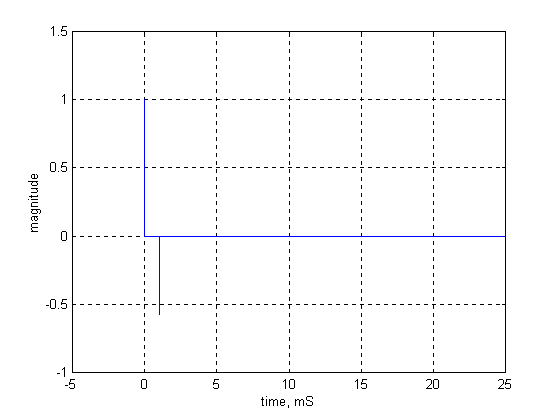Figure 4. Frequency domain behavior of a point source mounted in the center of the face of a long cylinder. Cylinder has a radius of 1/3 meter and measurement is taken far from the cylinder face.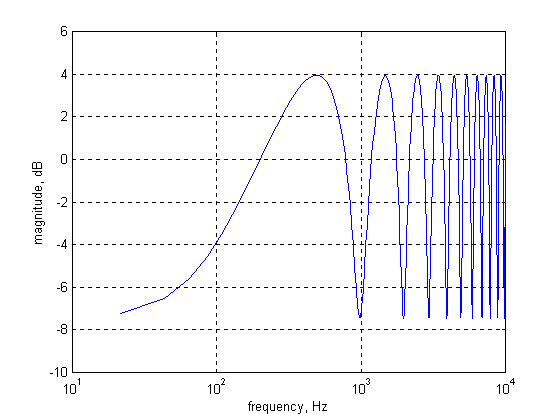Figure 5. Time domain behavior of a point source mounted on the center of a baffle the side of which is pi^.5 meters. Measurement is taken far from the face.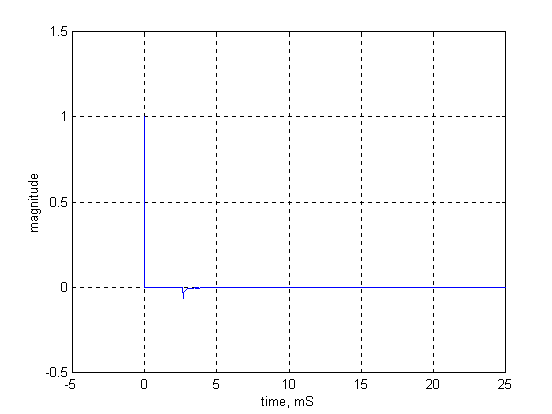Figure 6. Frequency domain behavior of a point source mounted on the center of a baffle the side of which is pi^.5 meters. Measurement is taken far from the face.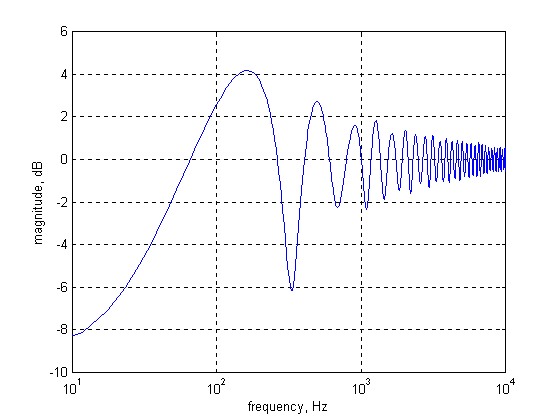Figure 7. Time domain behavior of a point source mounted on the center of a baffle with height of sqrt(pi)*4 and width of sqrt(pi)/4 meters. Measurement is taken far from the face.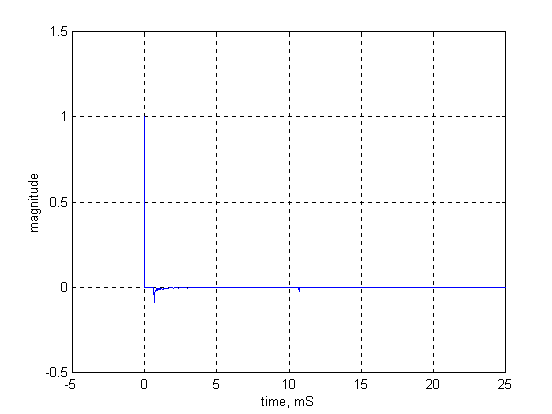Figure 8. Frequency domain behavior of a point source mounted on the center of a baffle with height of sqrt(pi)*4 and width of sqrt(pi)/4 meters. Measurement is taken far from the face.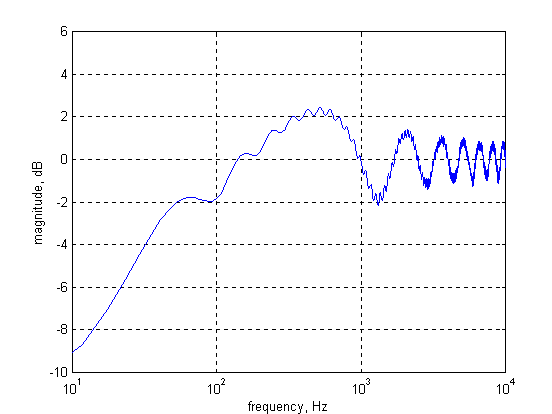Figure 9. Time domain behavior of a point source mounted in the upper left hand corner of a baffle with height of sqrt(pi)*4 and width of sqrt(pi)/4 meters. Measurement is taken far from the face.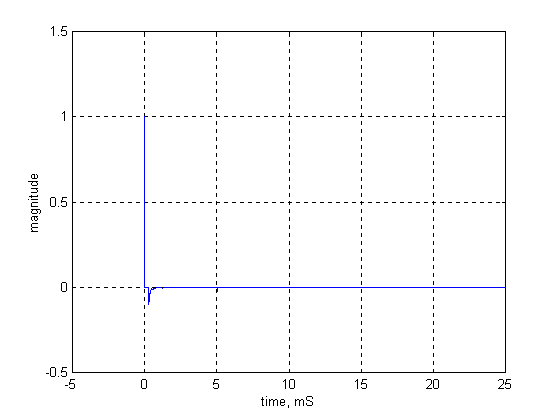Figure 10. Frequency domain behavior of a point source mounted in the upper left hand corner of a baffle with height of sqrt(pi)*4 and width of sqrt(pi)/4 meters. Measurement is taken far from the face.